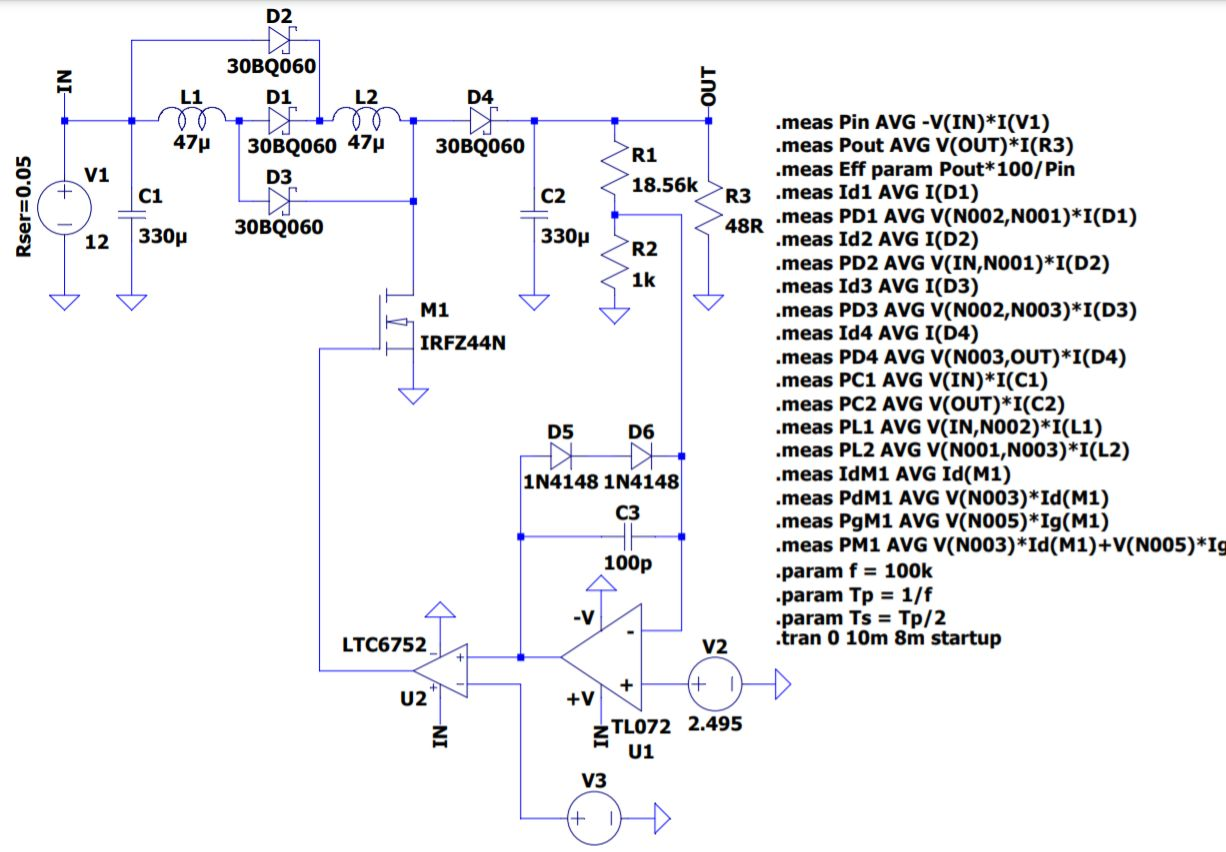1 year ago

Profile：.

• 579
• 0
• 0

Description

(Please find the LTspice simulation .asc file in the doccuments section.) A switched-inductor type boost converter has multiple inductors that are charged in parallel but discharged in series, thereby putting multiple boost inductors in series to the input power supply. The two diodes above and below the inductors enable parallel charging of the inductors when the MOSFET is on and the two diodes in series with the inductors put them in series to the input when the MOSFET is off. This results in an increased "boost" for the same duty cycle compared to a single inductor type boost converter.

The output voltage of a boost converter is given by the formula

Vo = Vin*n/(1 - D)

This circuit runs at a duty cycle of 65% and outputs 48V. A normal boost converter with an efficiency of 90% would output

Vo = 12*0.9/(1-0.65) = 30.85V

The output voltage of a two-inductor switched boost converter for the same duty cycle is

Vo = Vin*n*(1+D)/(1-D)

Substituting the appropriate values,

Vo = 12 *0.9*(1+0.65)/(1-0.65) = 50V

This circuit, in reality, outputs 48V with an efficiency of 92% (for a load current of 1A).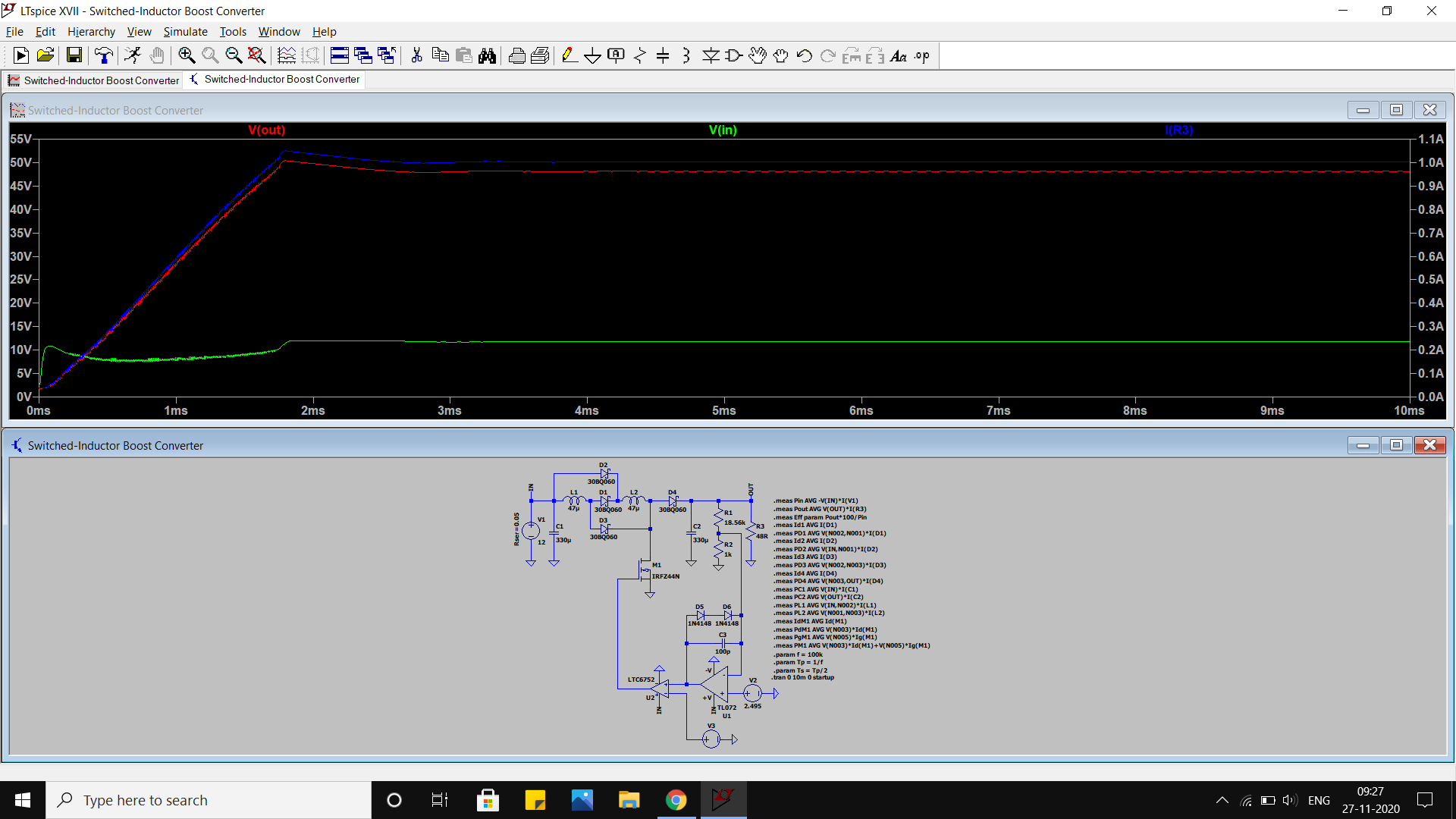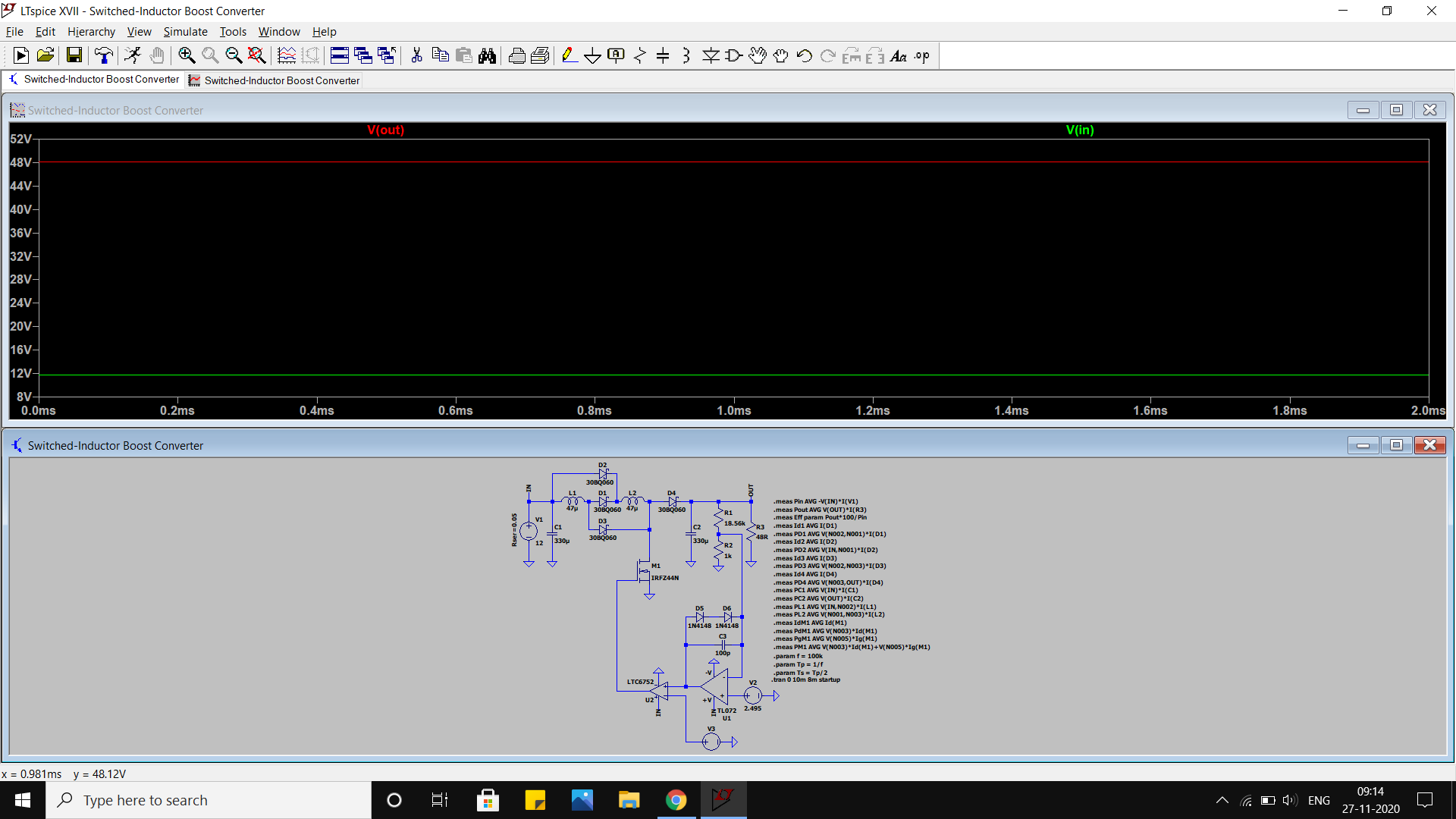Output voltage ripple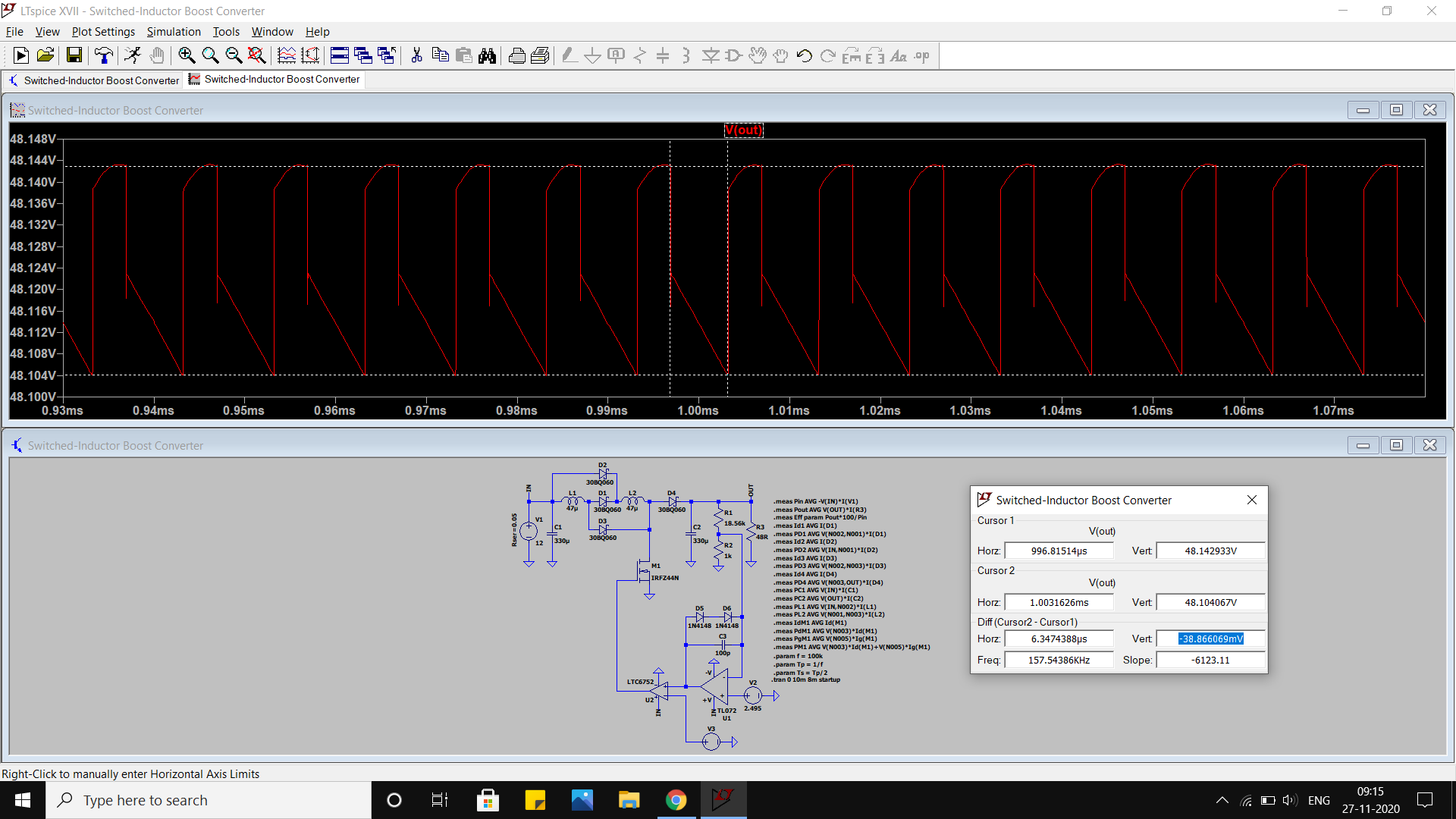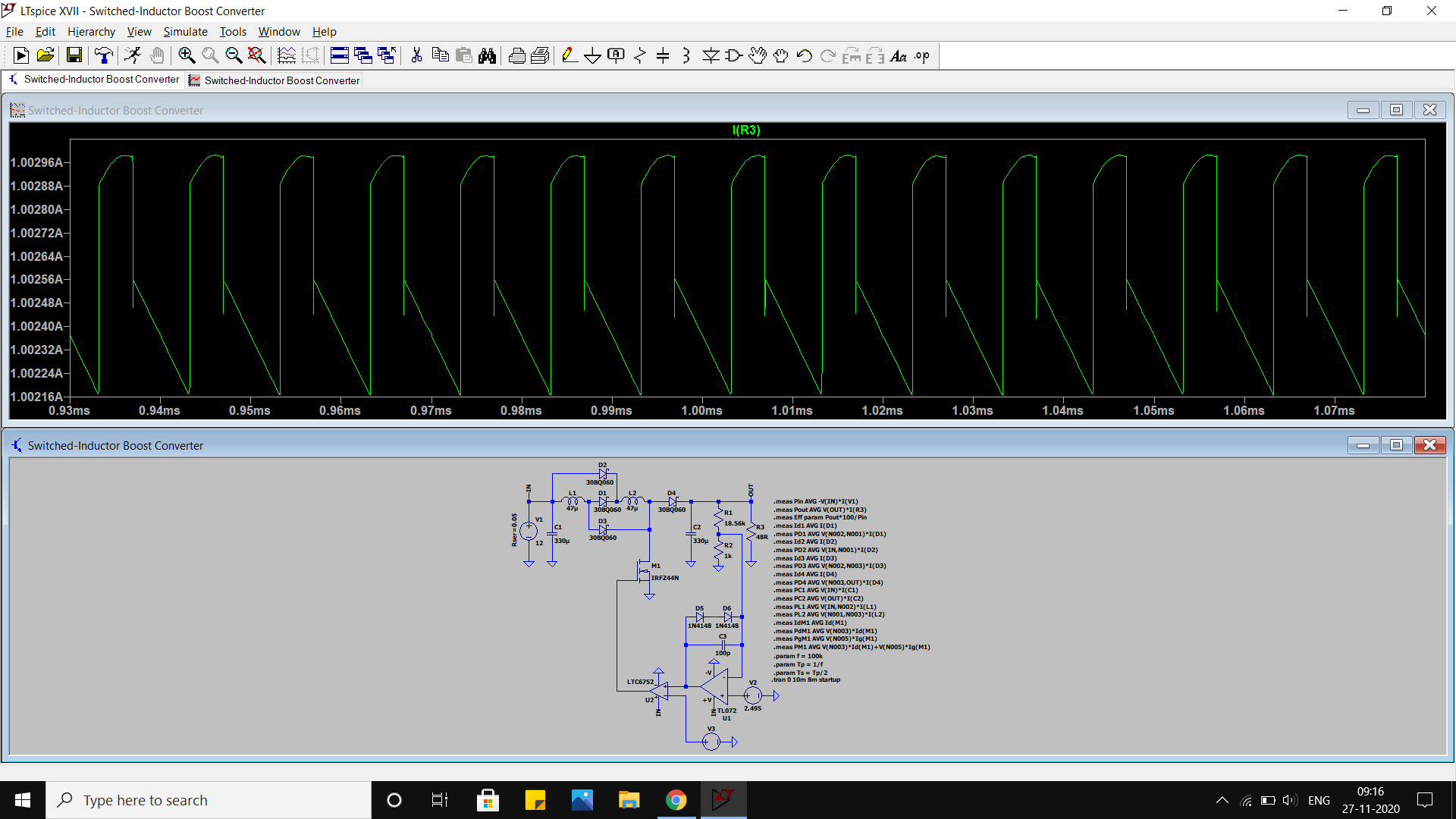PWM signal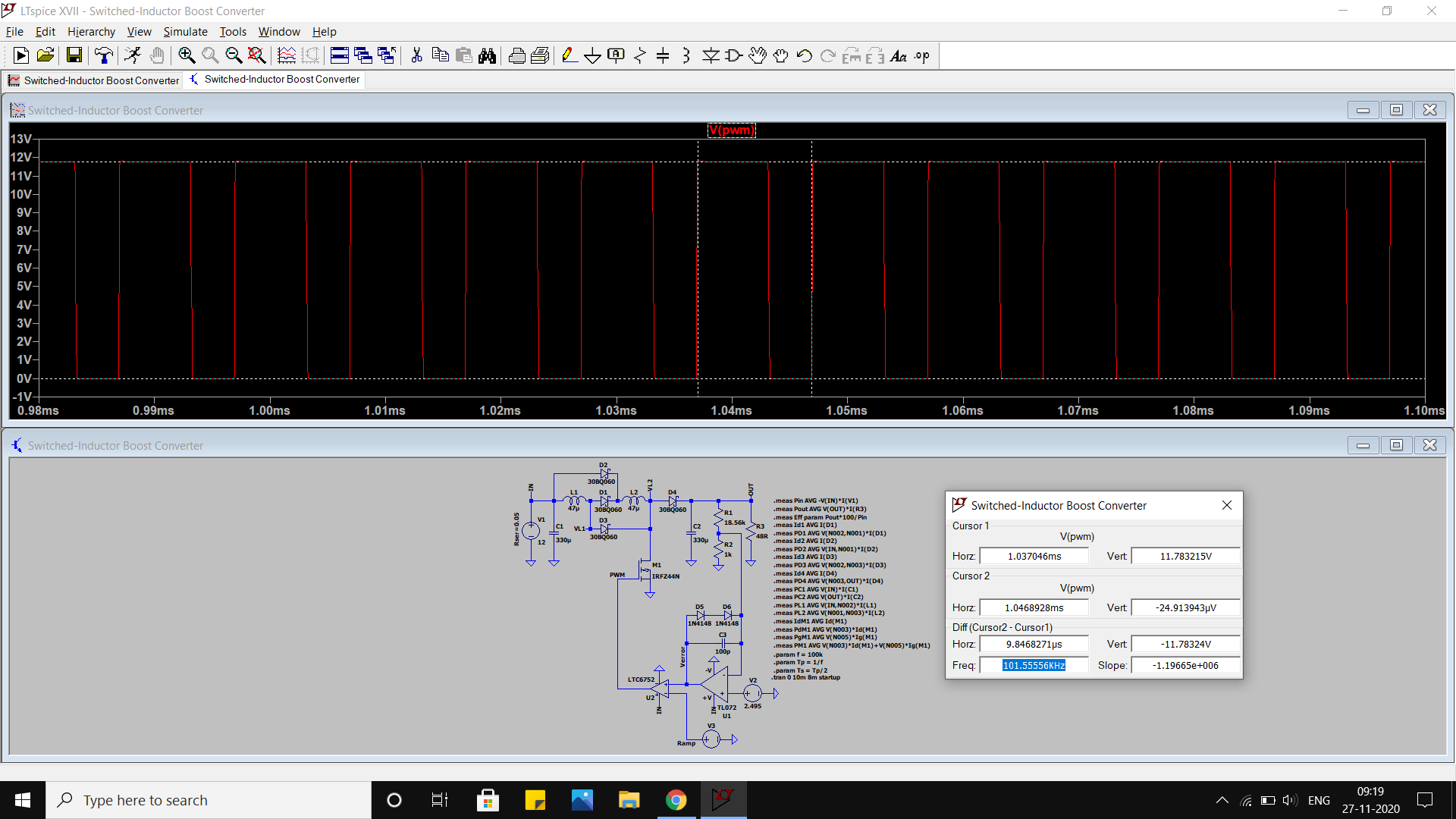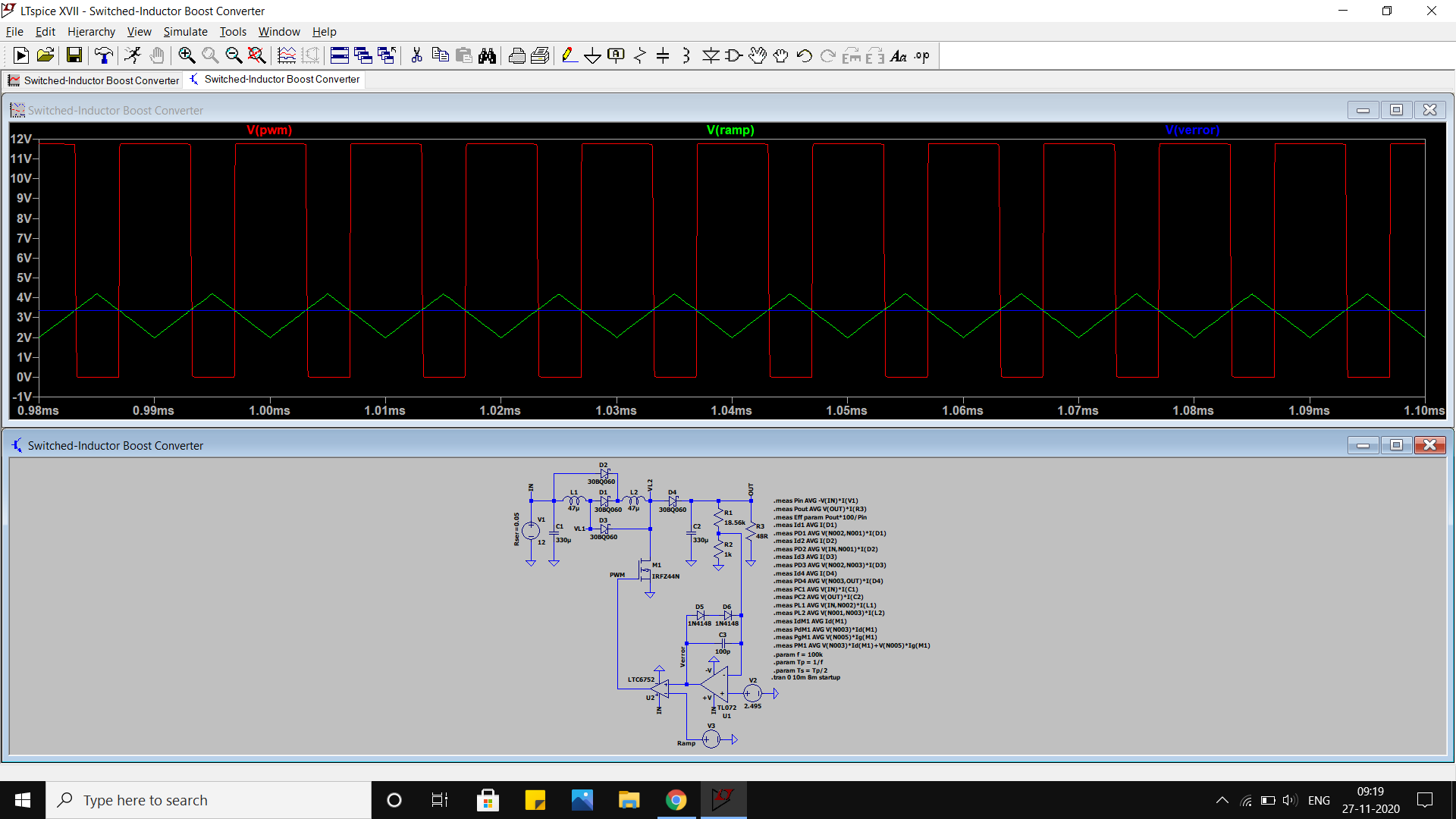Error voltage vs ramp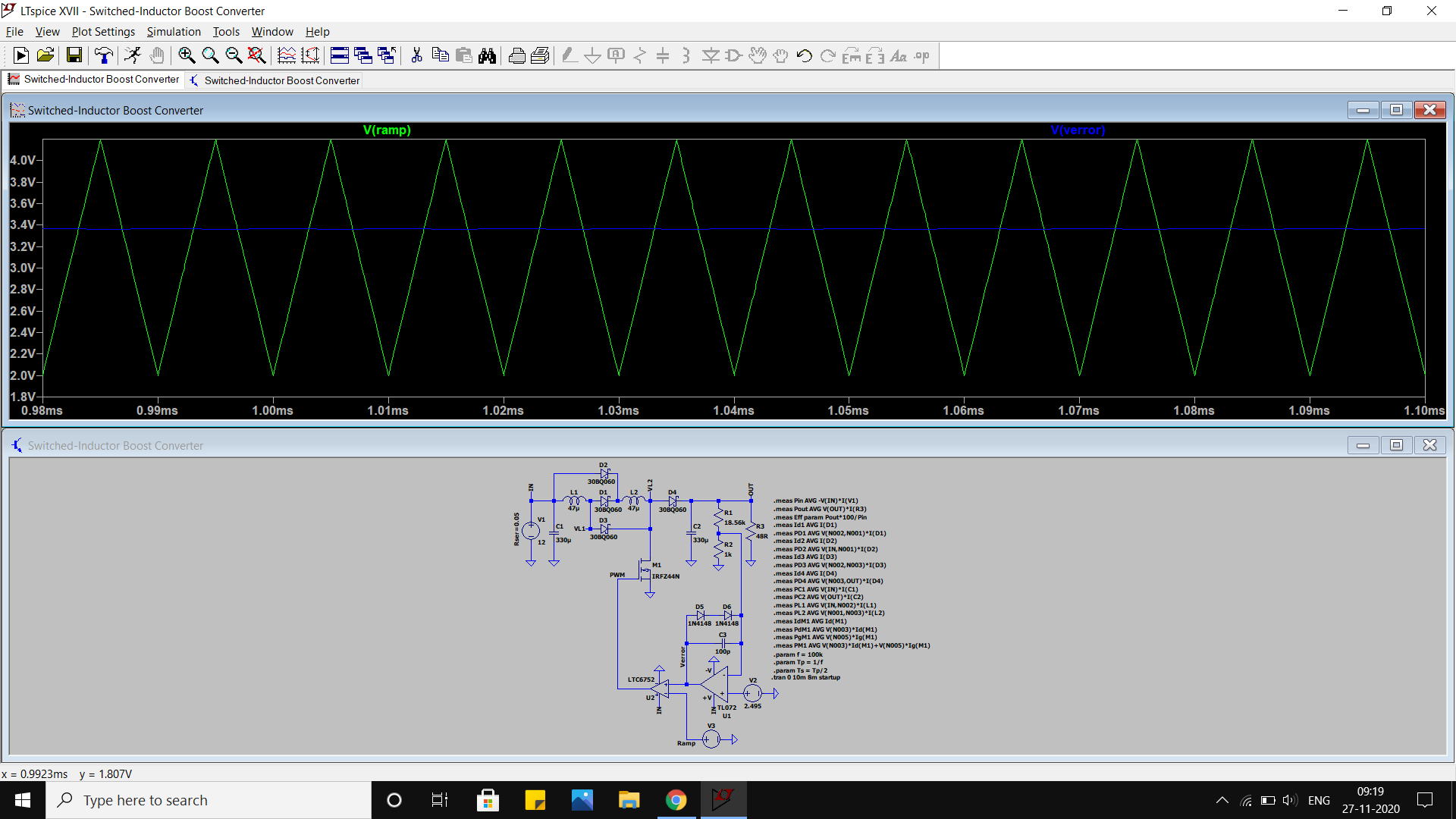Diode current waveforms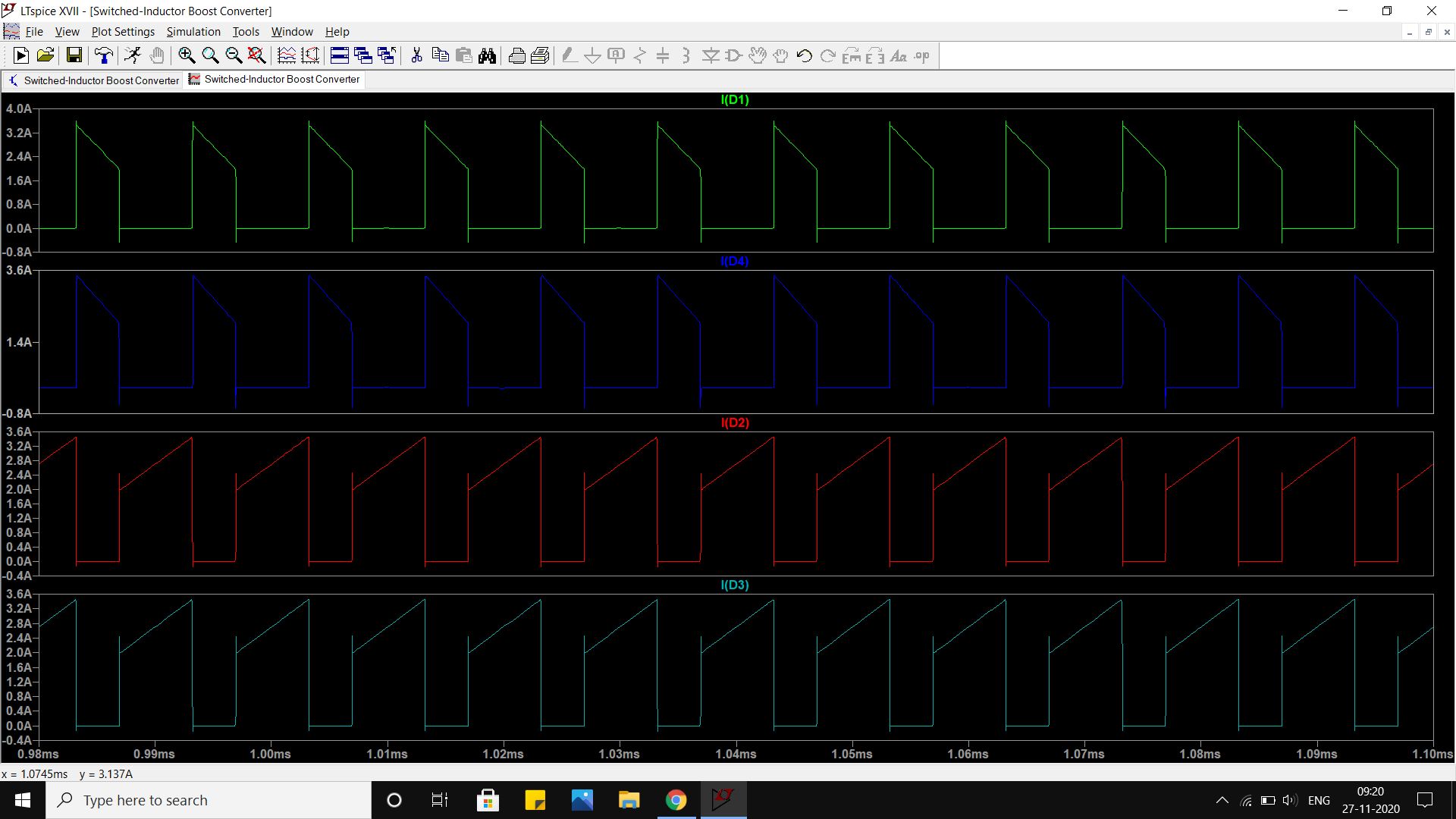Inductor and MOSFET voltages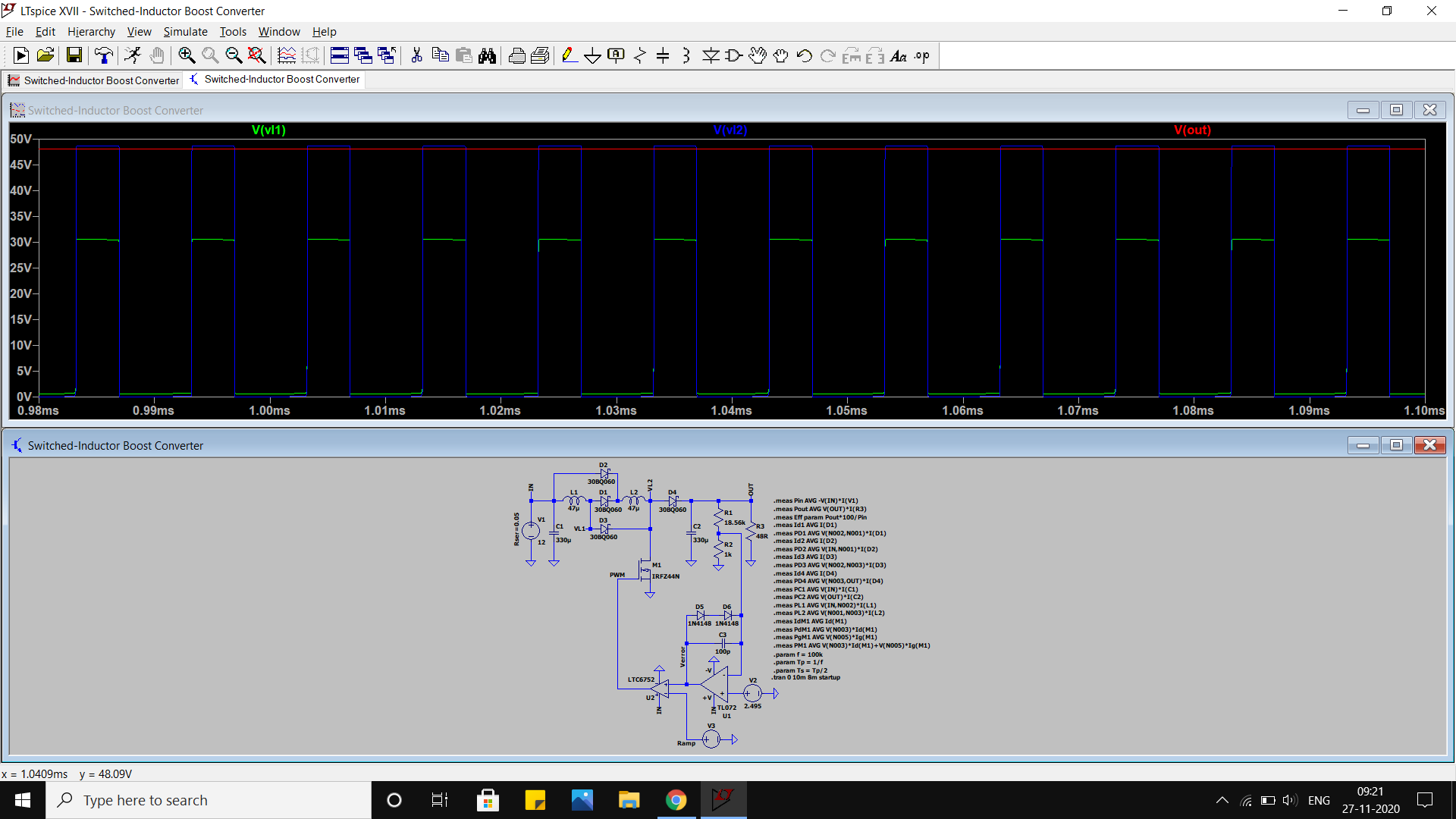Efficiecy and power losses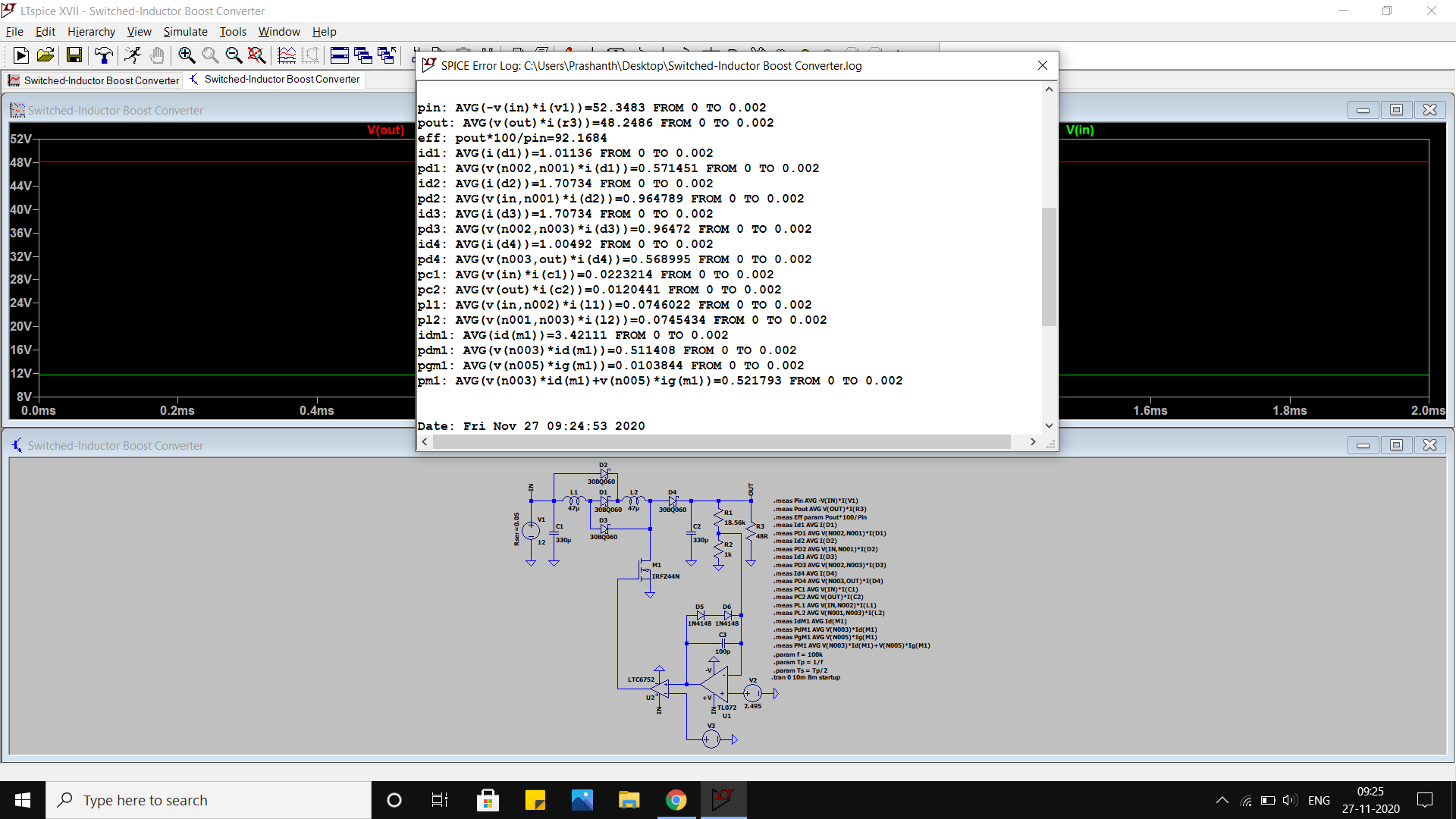Documents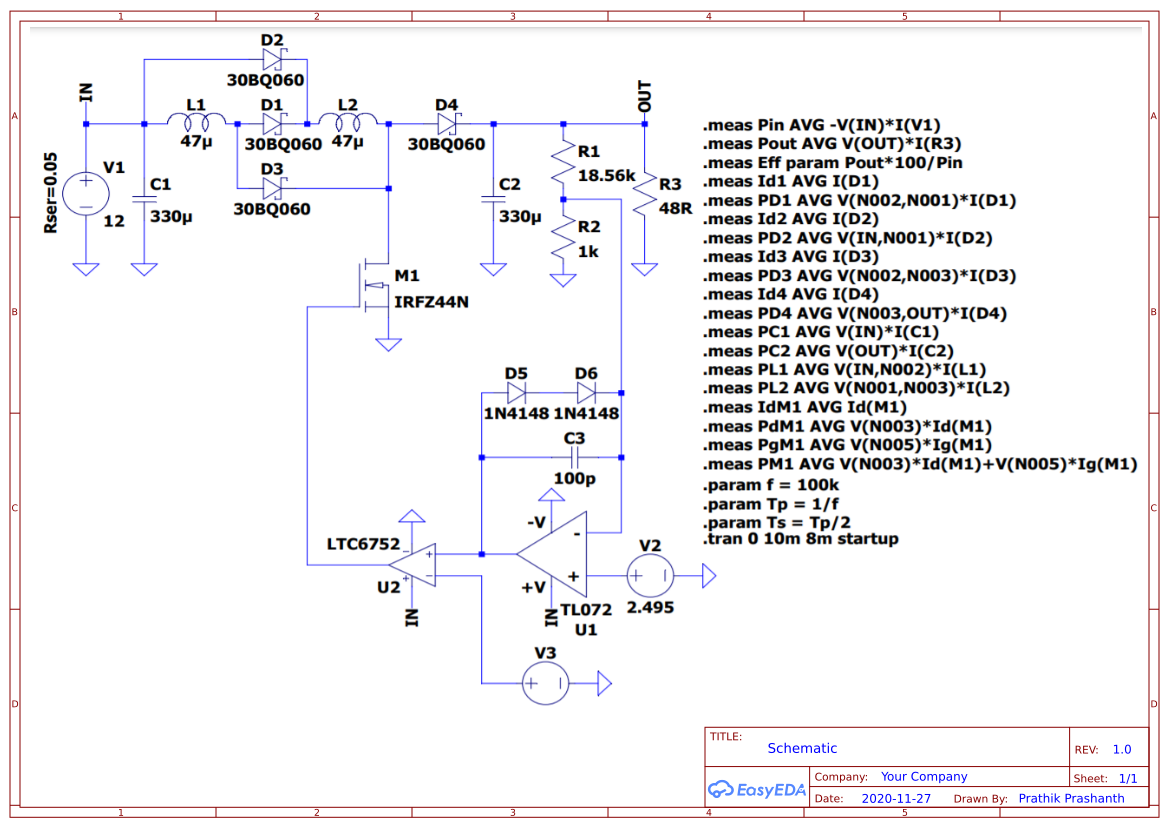Empty

Project Attachments• 0755 - 2382 4495
• 153 6159 2675

• 立创EDA微信号

easyeda• QQ交流群

664186054• 立创EDA公众号

lceda-cn